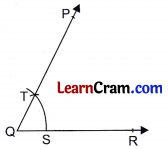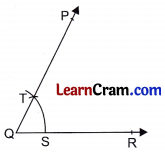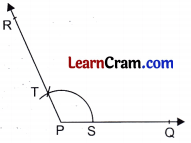# DAV Class 6 Maths Chapter 14 Worksheet 2 Solutions

The DAV Class 6 Maths Book Solutions and DAV Class 6 Maths Chapter 14 Worksheet 2 Solutions of Constructions offer comprehensive answers to textbook questions.

## DAV Class 6 Maths Ch 14 WS 2 Solutions

Question 1.
Draw an angle of any measure and name it. Using compasses and ruler, draw another angle equal to ∠A.Step 1: Draw an ∠BAC.
Step 2: With centre A and suitable radius, draw an arc which meets AC and AB at D and E respectively.
Step 3: Now draw a ray QR.
Step 4: Taking Q as centre and radius equal to AD, draw an arc which meets QR at S.
Step 5: Taking S as centre and radius equal to DE, draw an arc which cuts the first arc at T.
Step 6: Join QT and produce it to P.Hence, ∠PQR is the required angle equal to ∠A.

Question 2.
Draw an ∠XYZ equal to 90°. Using the compasses and ruler, draw YO the bisector of ∠XYZ. Measure ∠XYO and ∠ZYO. Are they equal?Step 1: Draw ∠XYZ = 90°
Step 2: Taking Y as centre and with suitable radius, draw an arc which meets YZ and XY at R and S respectively.
Step 3: Taking R and S as centres and radius equal to more than of SR, draw two arcs which intersects each other at T.
Step 4: Join YT and produce it to O.
Hence YO is the bisector of ∠XYZ such that ∠XYO = ∠ZYO = 45°Question 3.
Draw ∠CAB = 150° using protractor. Using compasses, draw another angle equal to ∠CAB.